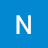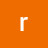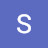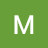Class 10 Maths ¦ Quadratic Equations ¦ Exercise 4.4 ¦ NCERT ¦ Maths TutorClass 10 Maths ¦ Quadratic Equations ¦ Exercise 4.4 ¦ NCERT ¦ Maths Tutor

Class 10 Maths ¦ Quadratic Equations ¦ Exercise 4.4 ¦ NCERT, CBSE,PSEB ¦ Maths Tutor ¦ Solutions from an experienced teacher ¦ Easiest explanation ¦

algebra tutors,Class 10,Class 10 maths,Class 10 ex 4.4 Q1,Class 10 maths exercise 4.4,Class 10 quadratic equations,Quadratic Equations class 10 ncert,Quadratic Equations exercise 4.4 class 10 q 1,10th math exercise 4.4 Q1,10th maths Quadratic Equations NCERT ex 4.4 Q2,Quadratic Equations class 10 cbse ex 4.4,Class 10 maths cbse exercise 4.4,Class 10 maths ex 4.4 cbse,Maths tutor,Class 10 maths ncert,Class 10 maths cbse ch 4 ex 4.4,exercise 4.4,Quadratic Equations

algebratutors.org

4 Responses to “Class 10 Maths ¦ Quadratic Equations ¦ Exercise 4.4 ¦ NCERT ¦ Maths Tutor”

1.NAVDEEP SINGH says:

Awsome video nice explaination

2.reena Maingi says:

Nice video

3.Sonu Anand says:

Good

4.Micky Uppal says:

Amazing video